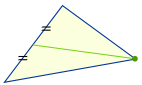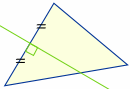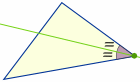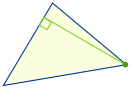# Triangle Centers

Where is the center of a triangle?

There are actually thousands of centers!

Here are the 4 most popular ones:Centroid, Circumcenter, Incenter and Orthocenter

For each of those, the "center" is where special lines cross, so it all depends on those lines!

Let's look at each one:

## CentroidDraw a line (called a "median") from each corner to the midpoint of the opposite side. Where all three lines intersect is the centroid, which is also the "center of mass":

Try this: cut a triangle from cardboard, draw the medians. Do they all meet at one point? Can you balance the triangle at that point?## CircumcenterDraw a line (called a "perpendicular bisector") at right angles to the midpoint of each side. Where all three lines intersect is the center of a triangle's "circumcircle", called the "circumcenter":

Try this: drag the points above until you get a right triangle (just by eye is OK). Where is the circumcenter? Why?

## IncenterDraw a line (called the "angle bisector") from a corner so that it splits the angle in half Where all three lines intersect is the center of a triangle's "incircle", called the "incenter":

Try this: find the incenter of a triangle using a compass and straightedge at: Inscribe a Circle in a Triangle

## OrthocenterDraw a line segment (called the "altitude") at right angles to a side that goes to the opposite corner. Where all three lines intersect is the "orthocenter":

Note that sometimes the edges of the triangle have to be extended outside the triangle to draw the altitudes. Then the orthocenter is also outside the triangle.